# 基于增量式非线性动态逆的四旋翼无人机姿态控制Incremental Nonlinear Dynamics Inversion for Attitude Control of Quadrotor

DOI: 10.12677/DSC.2018.74028, PDF, HTML, XML, 下载: 852  浏览: 2,136

Abstract: In view of the attitude control of quadrotor, a controller based on Incremental Control and Non-linear Dynamic Inversion (NDI) is designed to reduce the dependence of the traditional Dynamic Inversion control strategy on the model. Based on the Pixhawk open source control board, the angle and angular velocity control loop of the attitude control module is constructed by using the Nonlinear Dynamic Inversion (NDI) and Incremental Nonlinear Dynamic Inversion (INDI) control method. The stability and robustness of the nonlinear controller under external disturbances are verified through the software in the loop (SITL) simulation technology of PX4 firmware and Gazebo simulation software.

1. 引言

2. 四旋翼建模

2.1. 运动学方程

$R=\left[\begin{array}{c}\mathrm{cos}\left(\psi \right)\mathrm{cos}\left(\theta \right)\\ \mathrm{sin}\left(\psi \right)\mathrm{cos}\left(\theta \right)\\ -\mathrm{sin}\left(\theta \right)\end{array}\text{\hspace{0.17em}}\text{\hspace{0.17em}}\begin{array}{c}-\mathrm{sin}\left(\psi \right)\mathrm{cos}\left(\varphi \right)+\mathrm{cos}\left(\psi \right)\mathrm{sin}\left(\theta \right)\mathrm{sin}\left(\varphi \right)\\ \mathrm{cos}\left(\psi \right)\mathrm{cos}\left(\varphi \right)+\mathrm{sin}\left(\psi \right)\mathrm{sin}\left(\theta \right)\mathrm{sin}\left(\varphi \right)\\ \mathrm{cos}\left(\theta \right)\mathrm{sin}\left(\varphi \right)\end{array}\text{\hspace{0.17em}}\text{\hspace{0.17em}}\begin{array}{c}\mathrm{sin}\left(\psi \right)\mathrm{sin}\left(\varphi \right)+\mathrm{cos}\left(\psi \right)\mathrm{sin}\left(\theta \right)\mathrm{cos}\left(\varphi \right)\\ -\mathrm{cos}\left(\psi \right)\mathrm{sin}\left(\varphi \right)+\mathrm{sin}\left(\psi \right)\mathrm{sin}\left(\theta \right)\mathrm{cos}\left(\varphi \right)\\ \mathrm{cos}\left(\theta \right)\mathrm{cos}\left(\varphi \right)\end{array}\right]$ (1)

$\stackrel{˙}{P}=RV$ (2)

$\stackrel{˙}{\Omega }={R}_{w}\omega$ (3)

$P={\left(X,Y,Z\right)}^{\text{T}}$ 代表四旋翼的位置信息， $V={\left(U,V,W\right)}^{\text{T}}$ 是四旋翼在机体坐标系B下的线速度， $\Omega ={\left(\varphi ,\theta ,\psi \right)}^{\text{T}}$ 表示四旋翼的姿态角， $\omega ={\left(P,Q,R\right)}^{\text{T}}$ 为四旋翼机体坐标系B下的角速度。则旋转运动学方程用公式(4)表示。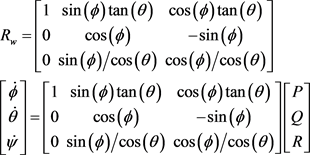(4)

2.2. 动力学方程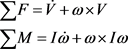(5)

I是四旋翼无人机关于质心的惯性张量矩阵，机体坐标系的原点Ob位于四旋翼的质心，由于四旋翼无人机为一刚体且关于X、Y轴对称，所以 ${I}_{xy}={I}_{xz}={I}_{yx}={I}_{yz}={I}_{zx}={I}_{zy}=0$ ，惯性矩阵可以转换为式(6)形式。

 (6)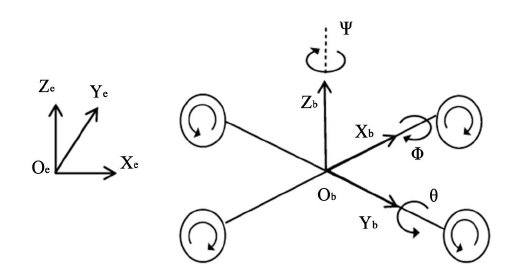Figure 1. The ground coordinate system E and the body coordinate system B

3. 动态逆控制器设计

3.1. 非线性动态逆

$\begin{array}{l}\stackrel{˙}{x}=f\left(x\right)+g\left(x\right)u\\ y=h\left(x\right)\end{array}$ (7)

$\stackrel{˙}{y}=\frac{\partial h}{\partial x}\stackrel{˙}{x}=\frac{\partial h}{\partial x}f\left(x\right)+\frac{\partial h}{\partial x}g\left(x\right)u=F\left(x\right)+G\left(x\right)u$ (8)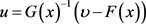(9)

3.2. 增量式非线性动态逆

$ẋ=f\left(x,u\right)$ (10)

$\begin{array}{l}\stackrel{˙}{x}\approx f\left({x}_{0},{u}_{0}\right)+{\frac{\partial f\left(x,u\right)}{\partial x}|}_{x={x}_{0},u={u}_{0}}\left(x-{x}_{0}\right)+{\frac{\partial f\left(x,u\right)}{\partial u}|}_{x={x}_{0},u={u}_{0}}\left(u-{u}_{0}\right)\\ \stackrel{˙}{x}\approx \stackrel{̇}{{x}_{0}}+F\left({x}_{0},{u}_{0}\right)\left(x-{x}_{0}\right)+G\left({x}_{0},{u}_{0}\right)\left(u-{u}_{0}\right)\end{array}$ (11)

$\stackrel{˙}{x}={\stackrel{˙}{x}}_{0}+G\left({x}_{0},{u}_{0}\right)\left(u-{u}_{0}\right)$ (12)

$u={u}_{0}+{G}^{-1}\left({x}_{0},{u}_{0}\right)\left(\upsilon -{\stackrel{˙}{x}}_{0}\right)$ (13)

${u}_{k+1}={u}_{k}+{G}^{-1}\left({x}_{k},{u}_{k}\right)\left({\upsilon }_{k+1}-\frac{{x}_{k}-{x}_{k-1}}{\Delta t}\right)$ (14)

${\upsilon }_{k+1}=\frac{{x}_{k+1}-{x}_{k}}{\Delta t}$ (15)

4. 四旋翼姿态控制器设计

4.1. 角度外环

$u={G}^{-1}\upsilon$ (16)

$\upsilon ={K}_{ang}e=\left[\begin{array}{c}{K}_{\varphi }\left({\varphi }_{d}-\varphi \right)\\ {K}_{\theta }\left({\theta }_{d}-\theta \right)\\ {K}_{\psi }\left({\psi }_{d}-\psi \right)\end{array}\right]$ (17)

${G}^{-1}=\left[\begin{array}{c}1\\ 0\\ 0\end{array}\text{\hspace{0.17em}}\begin{array}{c}0\\ \mathrm{cos}\left(\varphi \right)\\ -\mathrm{sin}\left(\varphi \right)\end{array}\text{\hspace{0.17em}}\begin{array}{c}-\mathrm{sin}\mathrm{s}\left(\theta \right)\\ \mathrm{cos}\left(\theta \right)\mathrm{sin}\mathrm{sin}\left(\varphi \right)\\ \mathrm{cos}\left(\theta \right)\mathrm{cos}\mathrm{cos}\left(\varphi \right)\end{array}\right]$ (18)是期望的欧拉角，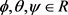是测量的欧拉角， ${K}_{ang}\in {R}^{3}$ 即为比例环节的增益。

4.2. 角速度内环

${\sum }^{\text{​}}M={M}_{a}+{M}_{c}$ (19)

${M}_{a}\in {R}^{3}$ 代表气动力距， ${M}_{c}\in {R}^{3}$ 表示系统的控制力距。在一个无穷小的时间间隔内，如果控制力据发生很小的变化 $\Delta {M}_{c}$ ，那么角加速度 $\stackrel{˙}{\omega }\in {R}^{3}$ 将随之变换，但是气动力距 ${M}_{a}$ 和角速度  并不会发生变化。这样，可以得到增量形式的表达式

$\Delta {M}_{c}=I\Delta \stackrel{˙}{\omega }=I\left({\stackrel{˙}{\omega }}_{d}-\stackrel{˙}{\omega }\right)$ (20)

$\Delta {M}_{c}$ 进行线性化并整理式(20)。

$\text{Δ}{M}_{c}={M}_{c}\left(\text{Δ}u\right)$ (21)

$\Delta u={M}_{c}^{-1}I\left(\upsilon -\stackrel{˙}{\omega }\right)$ (22)

$\upsilon ={K}_{vel}e=\left[\begin{array}{c}{K}_{p}\left({P}_{d}-P\right)\\ {K}_{q}\left({Q}_{d}-Q\right)\\ {K}_{r}\left({R}_{d}-R\right)\end{array}\right]$ (23)

${P}_{d},{Q}_{d},{R}_{d}\in R$ 是期望的角速度， $P,Q,R\in R$ 是测量的角速度， ${K}_{vel}\in {R}^{3}$ 是比例环节的增益。这样新的控制律中将不再有参数 ${M}_{a}$ ，不需要建立完整的气动力距方程，降低了对参考模型的依赖程度。图2是完整的姿态控制结构图。

5. 仿真结果

PX4固件支持软件在环仿真和硬件在环仿真两种仿真模式。软件在环仿真可以将完整的飞行控制栈运行在电脑上，通过仿真软件和地面站查看模拟飞行情况和飞行曲线，可以很好的验证改写代码的运行效果。硬件在环仿真是飞控板与仿真软件相连并将模拟固件运行在飞控板上的仿真，它可以测试代码在实际处理器中的运行结果。本文应用增量式非线性动态逆的控制策略对PX4固件进行改写，以Gazebo仿真软件为平台，搭建软件在环仿真的仿真环境，验证控制器在模拟飞行中的飞行效果，仿真数据流如图3所示。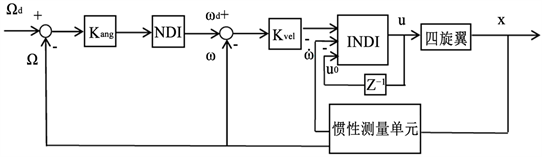Figure 2. Attitude control structure diagram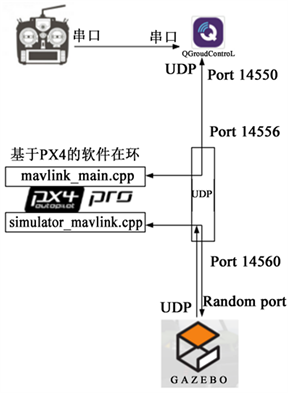Figure 3. Simulation data flow chart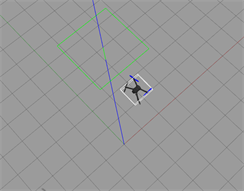Figure 5. Mission mode track map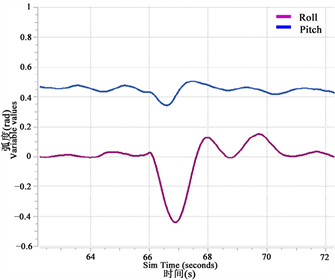Figure 6. The attitude curve of the 2nd navigation point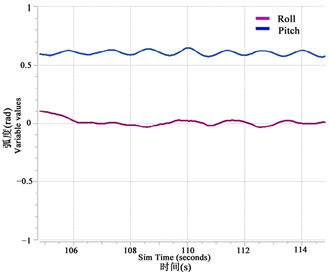Figure 7. The attitude curve of the 6th navigation point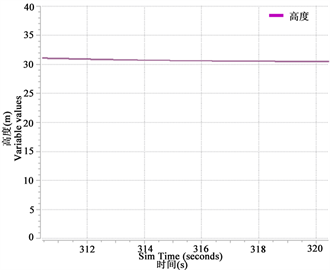Figure 8. Alt Hold mode attitude curve

6. 总结

  李一波, 李振, 张晓东. 无人机飞行控制方法研究现状与发展[J]. 飞行力学, 2011, 29(2): 1-5.  苏丙未, 万胜. 一种基于动态逆的控制方案在无人机中的应用研究[J]. 南京航空航天大学学报, 2000, 32(6): 706-710.  李怡勇, 沈怀荣. 无人机神经网络动态逆控制器设计研究[J]. 航天控制, 2009, 27(5): 3-6.  Weerdt, E.D., Kampen, E.V., Gemert, D.V., et al. (2008) Adap-tive Nonlinear Dynamic Inversion for Spacecraft Attitude Control with Fuel Sloshing. AIAA Guidance, Navigation and Control Conference and Exhibit. https://doi.org/10.2514/6.2008-7162  Acquatella, P.B., Falkena, W., Kampen, E.J.V., et al. (2012) Robust Nonlinear Spacecraft Attitude Control Using Incremental Nonlinear Dynamic Inversion. AIAA Guidance, Navigation, and Control Conference. https://doi.org/10.2514/6.2012-4623  Du, H., Pu, Z., Yi, J., et al. (2017) Advanced Quadrotor Takeoff Control Based on Incremental Nonlinear Dynamic Inversion and Integral Extended State Observer. Guidance, Navigation and Control Conference, IEEE, 1881-1886.  Smeur, E.J., Chu, Q.P. and Croon, G.C.D. (2016) Adaptive Incremental Nonlinear Dynamic Inversion for Attitude Control of Micro Aerial Vehicles. Journal of Guidance Control & Dynamics, 39, 1-12. https://doi.org/10.2514/1.G001490